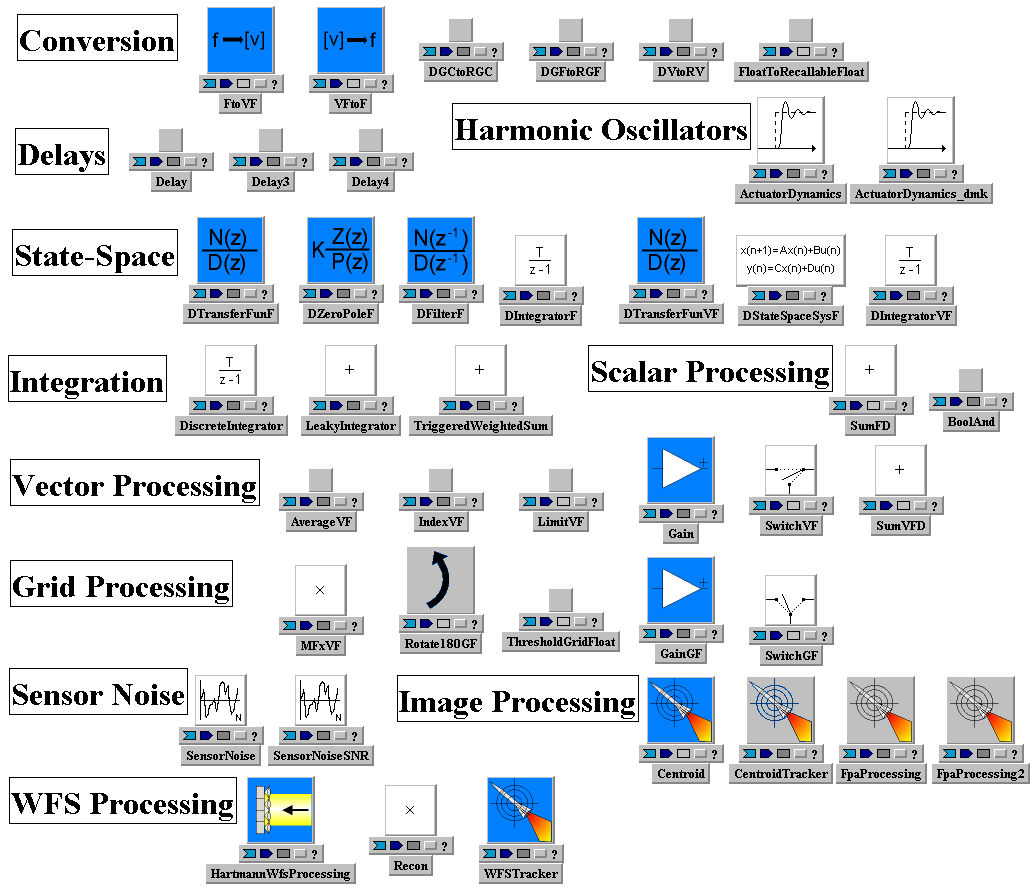tempusSystem Class ControlsLib
ControlsLib is part of the WaveTrain system class library

ControlsLib is one of six sub-libraries which make up WtLib, the WaveTrain component library. It contains components used in modeling control loops, including a number of special purpose components used in modeling adaptive optics and optical track loops. It also contains utilities for processing and converting between various types of WaveTrain data.Parameters
None
Inputs
None
Outputs
None
Subsystems

 ActuatorDynamics    actuatordynamics
 nact int timeLimit double timeConstant float pos0 Vector Vector() vel0 Vector Vector() acc0 Vector Vector()

 ActuatorDynamics_dmk    actdyn_dmk
 nact int d float m float k float timeLimit double 2.0*(range/speedOfLight)+0.001 pos0 Vector Vector() vel0 Vector Vector() acc0 Vector Vector()

 Centroid    centroid

 Recon    recon
 dmModel DMModel&

 Delay    delay
 delay float 0

 Delay3    delay3
 delay float 0

 DiscreteIntegrator    discreteintegrator
 startTime double 0.0 delta_t double 1.0 method integrationMethod FORWARD_EULER

 Gain    gain
 k float 1

 TriggeredWeightedSum    summer
 weight1 float 1 weight2 float 1

 FpaProcessing    fpaprocessing
 nx int ny int dx float dy float relativeThreshold float typeOfThreshold int n_per_average int 0

 HartmannWfsProcessing    hartmannwfsprocessing
 focalLength float magnification float xSubap Vector ySubap Vector centroidWidth float relativeThreshold float 0.1 absoluteThreshold float 0.0 xSlope0 float 0.0 ySlope0 float 0.0

 SensorNoise    sensornoise
 detectorGrid GridGeometry wavelength float quantumEfficiency float 1.0 rmsSignalIndependentNoise float background Grid responsivity Grid maxCount int addNoise bool false iseed int -123456789

 DZeroPoleF    dzeropolef
 zeros Vector vectorFloat(1,1.0) poles Vector vectorFloat(2,1.0,0.5) complexZeros Vector Vector() complexPoles Vector Vector() gain float 1 sampleTime double 1 priority int 0

 DFilterF    dfilterf
 numerator Vector vectorFloat(1,1.0) denominator Vector vectorFloat(2,1.0,0.5) sampleTime double 1

 DTransferFunF    dtransferfunf
 numerator Vector vectorFloat(1,1.0) denominator Vector vectorFloat(2,1.0,0.5) sampleTime double 1 priority int 0

 FtoVF    ftovf

 VFtoF    vftof

 SumVFD    sumvfd

 SumFD    sumfd

 DIntegratorVF    dintegratorvf
 sampleTime double 1 priority int 0

 DIntegratorF    dintegratorf
 sampleTime double 1 priority int 0

 DStateSpaceSysF    dstatespacesysf
 A Array arrayFloat(1,1,1.0) B Array arrayFloat(1,1,1.0) C Array arrayFloat(1,1,1.0) D Array arrayFloat(1,1,1.0) x0 Vector Vector(1,0.0) sampleTime double 1 priority int 0

 FloatToRecallableFloat    floattorecallablefloat

 DVtoRV    dvtorv
 timeLimit double 0

 LeakyIntegrator    leakyintegrator
 startTime double 0.0 delta_t double 1.0 method integrationMethod FORWARD_EULER alpha float 1.0 beta float 1.0

 AverageVF    averagevf
 vwavg0 float 0

 IndexVF    indexvf
 indeces Vector Vector() v0 Vector Vector()

 MFxVF    mfxvf
 mf Array Array

 SwitchVF    switchvf

 LimitVF    limitvf

 WFSTracker    wfstracker
 dmModel DMModel& DMModel() avgslopes0 Vector ZeroVecF(2)

 BoolAnd    switchvf2
 binit bool false

 CentroidTracker    centroidtracker
 centroid0 Vector Vector()

 SensorNoiseSNR    sensornoisesnr
 detectorGrid GridGeometry wavelength float quantumEfficiency float 1.0 rmsSignalIndependentNoise float background Grid responsivity Grid maxCount int addNoise bool false iseed int -123456789 relThreshSNR float 0.05 clipNegative bool true

 Delay4    delay4
 delay float 0.0 n int

 DGCtoRGC    dgctorgc
 timeLimit double 0

 DGFtoRGF    dgftorgf
 timeLimit double 0

 DTransferFunVF    dtransferfunvf
 numerator Vector vectorFloat(1,1.0) denominator Vector vectorFloat(2,1.0,0.5) sampleTime double 1 priority int 0

 FpaProcessing2    fpaprocessing2
 nx int ny int dx float dy float relativeThreshold float typeOfThreshold int n_per_average int 0

 GainGF    gaingf
 k float 1

 Rotate180GF    rotate180gf

 SwitchGF    switchgf

 ThresholdGridFloat    thresholdgridfloat

Last Saved: Tue Nov 03 11:13:24 MST 1998 by TVE version 2007B